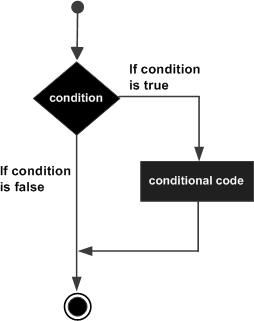# Pascal - if-then Statement

#### Learn Pascal Programming from Scratch

94 Lectures 8.5 hours

The if-then statement is the simplest form of control statement, frequently used in decision making and changing the control flow of the program execution.

## Syntax

Syntax for if-then statement is −

```if condition then S
```

Where condition is a Boolean or relational condition and S is a simple or compound statement. Example of an if-then statement is −

```if (a <= 20) then
c:= c+1;
```

If the boolean expression condition evaluates to true, then the block of code inside the if statement will be executed. If boolean expression evaluates to false, then the first set of code after the end of the if statement (after the closing end;) will be executed.

Pascal assumes any non-zero and non-nil values as true, and if it is either zero or nil, then it is assumed as false value.

## Flow Diagram### Example

Let us try a complete example that would illustrate the concept −

```program ifChecking;

var
{ local variable declaration }
a:integer;

begin
a:= 10;
(* check the boolean condition using if statement *)

if( a < 20 ) then
(* if condition is true then print the following *)
writeln('a is less than 20 ' );
writeln('value of a is : ', a);
end.
```

When the above code is compiled and executed, it produces the following result −

```a is less than 20
value of a is : 10
```
pascal_decision_making.htm